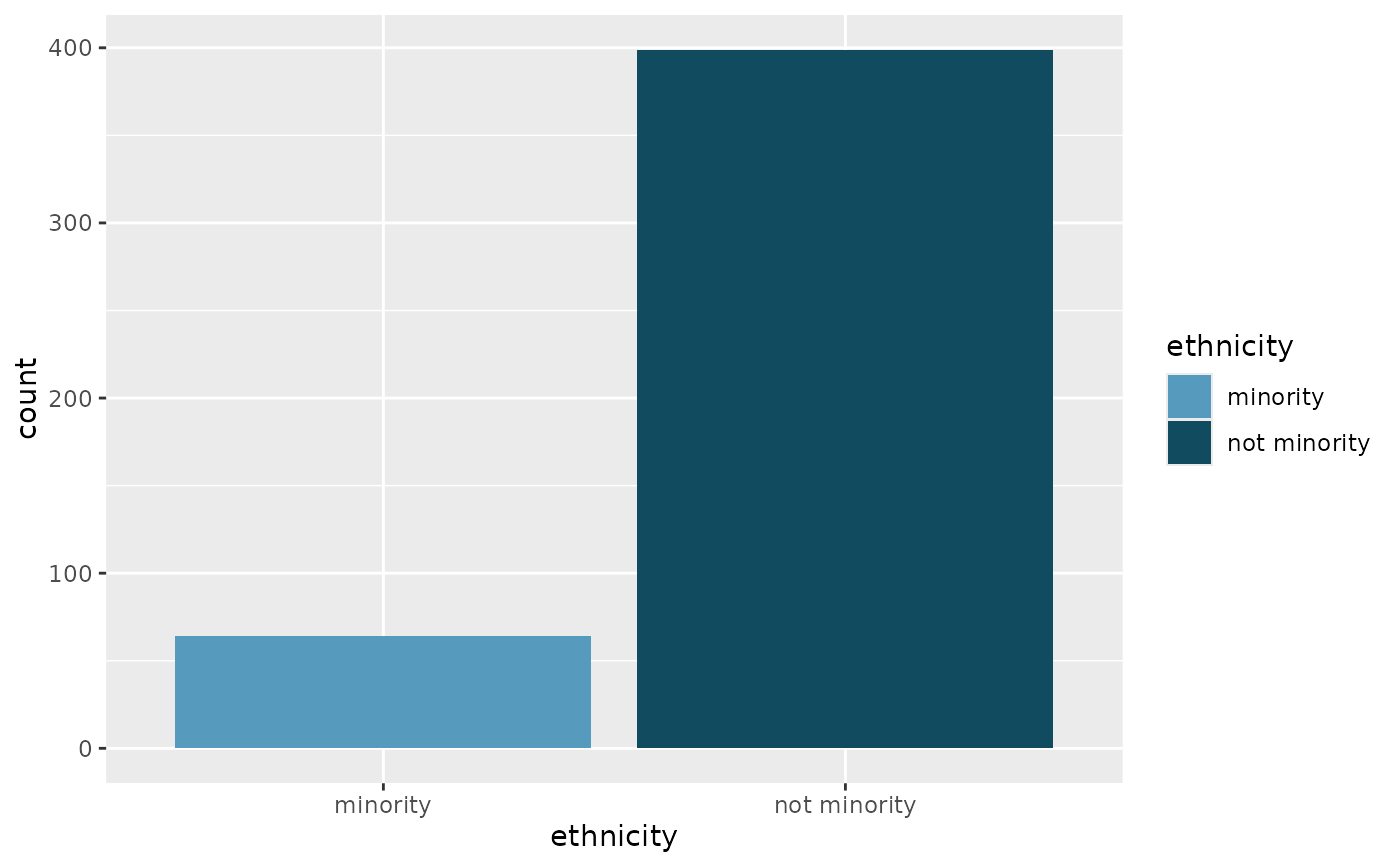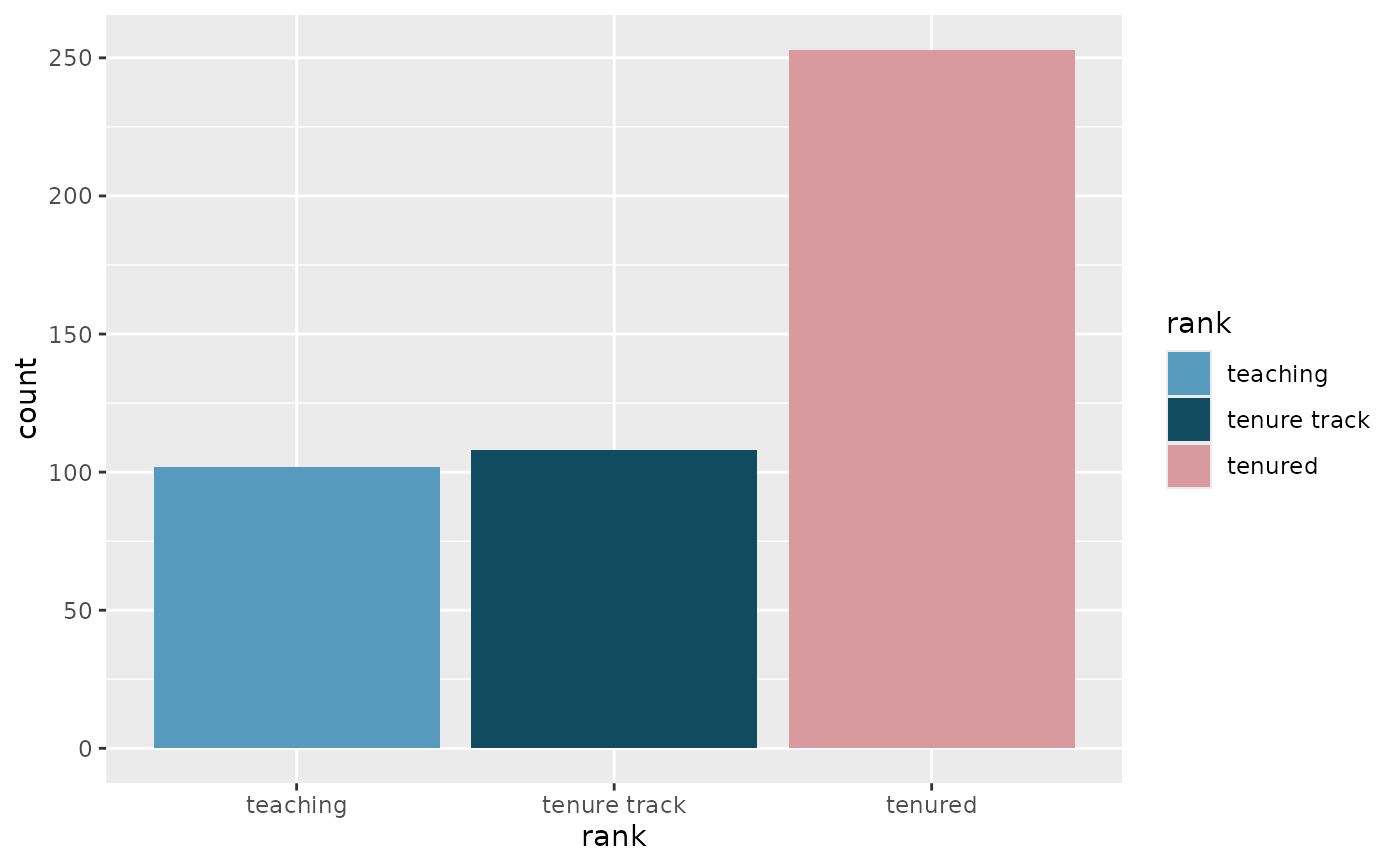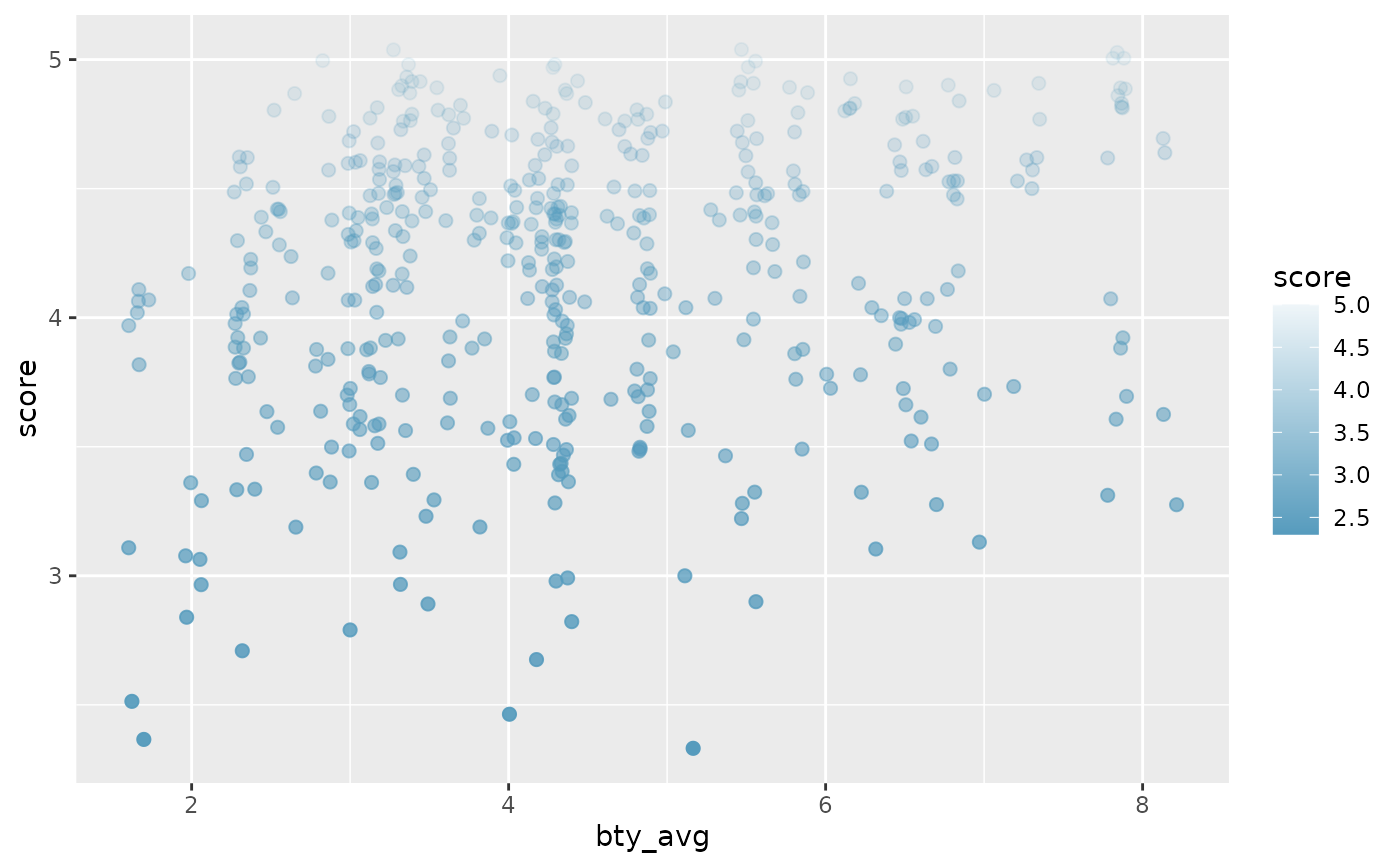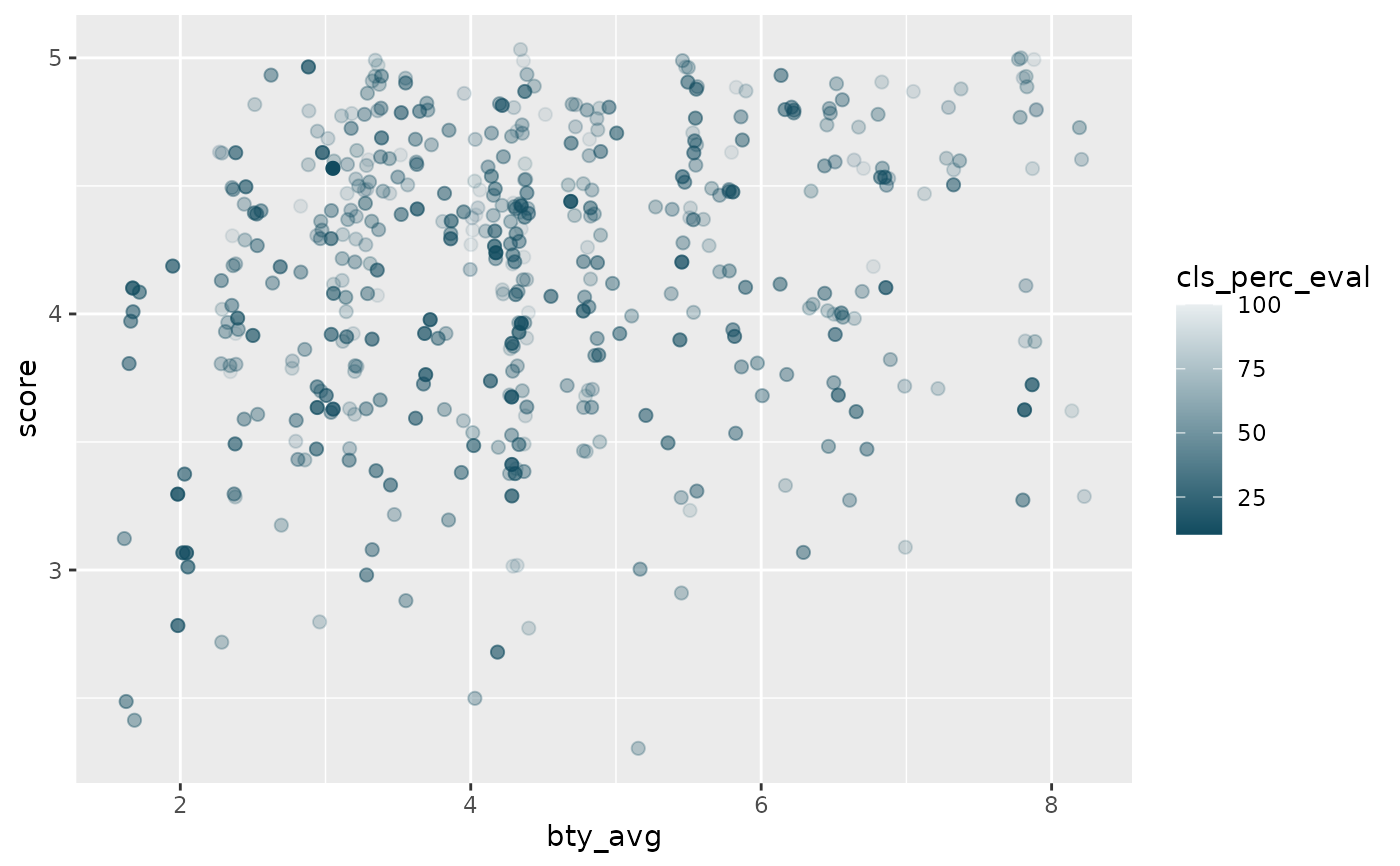Fill scale constructor for OpenIntro IMS colors

## Usage

scale_fill_openintro(palette = "main", discrete = TRUE, reverse = FALSE, ...)

## Arguments

palette

Character name of palette in openintro_palettes

discrete

Boolean indicating whether color aesthetic is discrete or not

reverse

Boolean indicating whether the palette should be reversed

...

Additional arguments passed to ggplot2::discrete_scale() or ggplot2::scale_fill_gradientn(), used respectively when discrete is TRUE or FALSE

## Examples


library(ggplot2)
library(dplyr)

# Categorical variable with two levels
ggplot(evals, aes(x = ethnicity, fill = ethnicity)) +
geom_bar() +
scale_fill_openintro("two")# Categorical variable with three levels
ggplot(evals, aes(x = rank, fill = rank)) +
geom_bar() +
scale_fill_openintro("three")# Continuous variable with levels
# Generates a palette, but may not be the best palette
# in terms of color-blind and grayscale friendliness
ggplot(diamonds, aes(x = clarity, fill = clarity)) +
geom_bar() +
scale_fill_openintro()# For continuous palettes
ggplot(evals, aes(
x = bty_avg, y = score,
color = score
)) +
geom_jitter(size = 2) +
scale_color_gradient(low = IMSCOL["blue", "full"], high = IMSCOL["blue", "f6"])ggplot(evals, aes(
x = bty_avg, y = score,
color = cls_perc_eval
)) +
geom_jitter(size = 2) +
scale_color_gradient(low = IMSCOL["green", "full"], high = IMSCOL["green", "f6"])